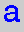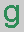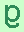The ABC of DNA Computing

For web information about DNA consult the Human Genome Project page at Oak Ridge. For information about NP-complete problems try Forbes Lewis' page at the University of Kentucky. A useful print reference is DNA Computing by Paun, Rozenberg and Salomaa (Springer, 1998).

## 1. What is DNA?

DNA (Deoxyribonucleic acid) is the molecular basis of genetics. For our purposes, the following features are important.
• A DNA molecule is made of two intertwined, parallel strands (the ``double helix'').

• Each strand has the following structure:
```
p   p   p             p = phosphate
.. \ / \ / \ / \ / ...
s   s   s   s           s = sugar
|   |   |   |
b   b   b   b           b = base
```
where each b can be any one of four bases:= adenine,= thymine,= cytosine,= guanine.
• Two consecutive s-p bonds must occur at distinct places on the s molecule: one at the 3' (``three-prime'') position and one at the 5', so each strand has a 3'-end and a 5'-end, and so strands can be systematically oriented. We will write,,,when the strand is being read from the 3'-end to the 5'-end, and,,,when the strand is being read the opposite way, that is, from the 5'-end to the 3'-end.
• The two intertwined strands in a DNA molecule have opposite orientations, and complementary base sequences. This means that opposite everyon one strand is aon the other, and opposite everyis an. Likewise opposite everyon one strand is aon the other, and opposite everyis a. A typical stretch of the DNA molecule looks like:
 ``` p p p p ... 3'\ / \ / \ / \ / \ /5' ... s s s s s | | | | || | | | || | | | | s s s s s ... 5'/ \ / \ / \ / \ / \3' ... p p p p ``` In what follows, this schematic representation of a segment of a DNA molecule will be abbreviated to:Complementarity, the fundamental key to DNA replication, is also the basic mathematical ingredient in DNA computing. --Tony Phillips
SUNY at Stony Brook